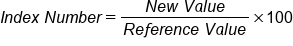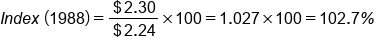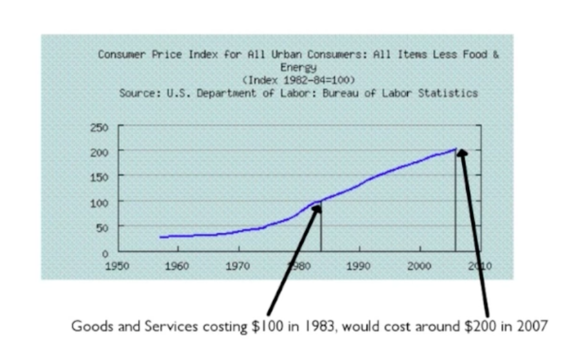+

# Index Number and Reference Value

Author: Sophia Tutorial
##### Description:

Identify index numbers from a reference value.

(more)

Sophia’s self-paced online courses are a great way to save time and money as you earn credits eligible for transfer to many different colleges and universities.*

No credit card required

46 Sophia partners guarantee credit transfer.

299 Institutions have accepted or given pre-approval for credit transfer.

* The American Council on Education's College Credit Recommendation Service (ACE Credit®) has evaluated and recommended college credit for 33 of Sophia’s online courses. Many different colleges and universities consider ACE CREDIT recommendations in determining the applicability to their course and degree programs.

Tutorial
what's covered
This tutorial is going to teach you about index numbers and reference values, through the definition and discussion of:

1. Index Numbers and Reference Values
2. Consumer Price Index and Inflation

## 1. Index Numbers and Reference Values

An index number is a way to measure a percent increase or decrease from one point to another. This is typically done with price changes. We set an arbitrary starting point in time and assign that price an index number of 100. This starting price is called the reference value because we refer back to it every time the price changes.

To calculate the index value for other points in time, you would take the current price, divide by the reference value, and then convert that value to a percent.

formula
Index NumberHow do we work with index numbers and reference values most of the time? Consider the following example:

In 1983 a gallon of milk cost \$2.24, so you assign this reference value of \$2.24 an index value of 100. Essentially this means that it cost 100% of what it cost in 1983--a fairly obvious statement.

 Year 1983 1988 1993 1998 2003 Price(\$) \$2.24 \$2.30 \$2.86 \$3.16 \$3.19 Index Value 100

To calculate the index value for other points in time, like in 1988 when a gallon of milk costs \$2.30 or 1993 when it cost \$2.86, you would take the current price, divide by the reference value of \$2.24, and then convert that value to a percent.The index value in 1998, then, is \$2.30 divided by the reference value of \$2.24. That gives you 1.027, which as a percent is 102.7%. Note that index values are expressed without the percent symbol, so the index value in 1988 was 102.7. You can complete the table with the remaining values.

 Year 1983 1988 1993 1998 2003 Price(\$) \$2.24 \$2.30 \$2.86 \$3.16 \$3.19 Index Value 100 102.7 127.7 141.1 142.4

What this indicates is that by the time you get to 2003, a gallon of milk cost 142.4% as much as it did in 1983, or a 42% increase over 1983.

terms to know

Index Number
A way to measure the relative change in a value, usually the price of a good or service, over time. If the index number is over 100, that means the price has increased. If the price has decreased, then the index number will be less than 100.
Reference Value
An arbitrarily chosen starting value for an index. It is assigned an index number of 100.

## 2. Consumer Price Index and Inflation

The most prominent index number that you see in everyday life is called the Consumer Price Index. The Consumer Price Index (abbreviated as CPI) measures a percent increase or decrease in the price of goods and services. Its reference value is 1983, which is why that was the reference value used in the previous example. The U.S. Bureau of Labor Statistics updates the CPI every month.

The CPI is a general measure of inflation. Inflation means that the index is going up. It's a decline in purchasing power, which means that it costs more now to buy these goods and services than it did then. That means that the dollar is inflated. Put another way, inflation means that with the same amount of money coming in and with the same income, you have less purchasing power. It may cost you much more now to do what it cost \$100 to do in 1983.

Here's a graph of the CPI over time. Notice the index value is 100 in 1983, between 1980 and 1990. Goods and services costing \$100 in 1983 will cost you around \$200 if you look at around 2007. Therefore, the index value was 200 in 2007.terms to know

Consumer Price Index
An index published by the US Bureau of Labor Statistics that shows the change in the price of many different goods or services in the United States. It provides a measure of purchasing power.
Inflation
A relative increase in the price of a good or service over time. A person will need to pay more to receive the same good or service than they did at a previous point in time.

summary
Index numbers allow us to check changes, typically in prices, from one point in time to another. We begin with a reference value, which is the price at some arbitrary point in time. The index numbers are the percent increase or decrease from that reference value. If the price goes up, the index number will be over 100. If the price goes down, the index number will be under 100. The most commonly referred index would be the Consumer Price Index or CPI. The CPI shows percent increase or decrease in the prices of many goods and services, which helps determine the amount of inflation.

Good luck!

Source: Adapted from Sophia tutorial by Jonathan Osters.

Terms to Know
Consumer Price Index

An index published by the US Bureau of Labor Statistics that shows the change in the price of many different goods or services in the United States. It provides a measure of purchasing power.

Index Number

A way to measure the relative change in a value, usually the price of a good or service, over time. If the index number is over 100, that means the price has increased. If the price has decreased, then the index number will be less than 100.

Inflation

A relative increase in the price of a good or service over time. A person will need to pay more to receive the same good or service than they did at a previous point in time.

Reference Value

An arbitrarily chosen starting value for an index. It is assigned an index number of 100.

Formulas to Know
Index NumberRating